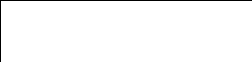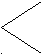# Sph4u electric Fields Multiple Choice

 SPH4U Electric Fields Multiple Choice Choose the best possible answer. Say: “Banana Phone”. 1) The number of electrons that must be collected on a sphere to give it a charge of –5.6 x 10-6 C is: A) 9.0 x 10-25 B) 2.9 x 10-14 C) 3.5 x 1013 D) 2.9 x 1014 2) Two very small conducting spheres with equal radii have charges 3.0 x 10-6 C and – 6.0 x 10-6 C. The spheres are touched together and then held 5.0 cm apart. What is the magnitude of the force between the spheres? A) 65 N B) 8.1 N C) 6.5 x 10-3 N D) 8.1 x 10-4 N 3) One coulomb is defined as the: A) Charge of one mole of electrons B) Force experienced by a unit of charge C) Charge that flows in one second when the current is one ampere D) Power produced when energy flows at a rate of one Joule per second 4) A Coulomb is a unit of: B) EMF C) Charge D) Current 5) Coulomb's law concerns itself with: A) The force exerted by one mass on another mass B) The interaction between two static charges C) The application of electric charge D) Conservation of electric charge 6) If the force between two charged particles is to remain the same after the charge on one particle is tripled and the charge on the other is increased to twelve times its original value, then the distance between the charges should be: A) 1/36 of the original value B) 1/6 of the original value C) 36 times the original value 7) If a small object with a charge of 10.0 C is located 5.0 m from another small object with a charge of 10.0 C, then the electrical force between them is: A) 1.8 x l010 N B) 2.4 x 1010 N C) 3.0 x 1010 N D) 3.6 x 1010 N 8) An electric field is: A) A field that carries an electrical charge B) An area of electrical influence surrounding a charge C) A field that will always cause uncharged objects to become charged D) A large grassy area where the electrons roam and graze 9) In the absence of other external forces, a charged particle in an electric field will: A) Experience an acceleration B) Be forced to move in a circular path C) Adjust its charge to maintain equilibrium with the field D) Remain stationary, dependent on its charge and the strength of the field 10) The strength of an electric field for point charges varies: A) Directly with distance B) Inversely with the distance C) Directly as the square of the distance D) Inversely as the square of the distance 11) The units of electric field intensity are: A) V/m and N/C B) C/m and C2/N C) V/C and V/J D) V/m2 and s2/C 12) The direction of an electric field is determined using: B) A negative test charge C) The flow of positive charge D) The flow of negative charge 13) If a negatively charged body is placed in an electric field that is directed down, the direction of the force on the body is: A) Right B) Left C) Up D) Down 14) The magnitude of the electric field exerting a force of 0.60 N on a charge of 40.0 pC is: A) 1.5 x 107 V/m B) 1.5 x 1010 V/m C) 6.7 x 10-11 V/m D) 6.7 x l0-8 V/m 15) The force on an electron in an electric field of magnitude 3.2 x 104 N/C is: A) 5.12 x 10-15 N B) 5.00 x 10-24 N C) 2.00 x 1023 N D) 1.60 x l0-15 N 16) The electric field strength 2.0 x 10-10 m from an alpha particle is: A) 7.2 x 1010 N/C B) 1.4 x l01 N/C C) 3.4 x 1010 N/C D) 8.2 x l010 N/C Use the following diagram to answer the next question.Three equal positive charges are located at the vertices of an equilateral triangle. P 17) The direction of the electric field at point P is: A) Into the page B) Out of the page C) West D) East 18) A unit of electric potential is the: A) Coulomb B) Volt C) Ampere D) Ohm 19) One Volt is: A) One Joule per Coulomb B) One Newton per Coulomb C) One Newton per Amp-meter D) One Joule per Watt 20) A proton is accelerated through a one megavolt potential. Its final speed is: A) 9.1 x 107 m/s B) 1.9 x l07 m/s C) 1.4 x 104 m/s D) 1.4 x 107 m/s 21) The electron-Volt is a unit of: A) Potential B) Charge C) Power D) Energy 22) An electron is moved through a P.D. of 2 V. The work done is: A) 4 J B) 2 eV C) 2 J D) ½ eV 23) Millikan decided the lowest charge he found was a one-electron charge as: A) This value agreed with that obtained by equating q|E| = mg B) All other values were multiples of this value C) When the mass was related to the force of gravity, this value was the minimum charge required D) This value agreed with JJ Thompson’s charge to mass ratio experiments E) This value agreed with the one Millikan cooked up 24) The minimum indivisible unit of charge is: A) One coulomb B) The charge of an alpha particle C) The charge on an electron D) All of the aboveDownload 28.98 Kb.Share with your friends: